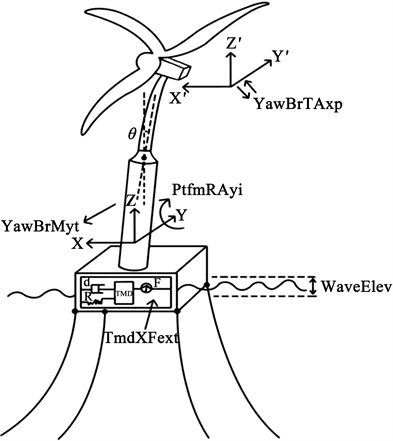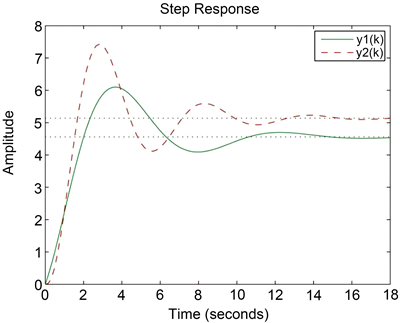#### 期刊菜单

Application of Finite-Frequency Model Reduction Method to Floating Wind Turbine
DOI: 10.12677/CSA.2021.1111278, PDF, HTML, XML, 下载: 19  浏览: 79

Abstract: This paper studies the model reduction of offshore floating wind turbine. Firstly, we use the measured data to construct the switched linear discrete time-delay model of offshore floating wind turbine. Secondly, we propose an index that can estimate the finite-frequency index, and introduce some relaxation matrices. These methods can obtain finite-frequency performance conditions and stability criteria, and realize the transformation of turbine model from high order to low order, which reduces the conservatism and computational complexity of the results. Finally, a numerical example is given to illustrate the effectiveness of the proposed method.

1. 引言

2. 风机模型设计及问题描述

$\left\{\begin{array}{l}x\left(k+1\right)={A}_{\sigma \left(k\right)}x\left(k\right)+{A}_{d\sigma \left(k\right)}x\left(k-d\right)+{B}_{\sigma \left(k\right)}u\left(k\right)\\ y\left(k\right)={C}_{\sigma \left(k\right)}x\left(k\right)+{C}_{d\sigma \left(k\right)}x\left(k-d\right)+{D}_{\sigma \left(k\right)}u\left(k\right)\\ x\left(k\right)=\varphi \left(k\right),\text{\hspace{0.17em}}\text{\hspace{0.17em}}\text{for}\text{\hspace{0.17em}}k=-d,-d+1,\cdots ,0\end{array}$ (1)

$\sigma \left(k\right):\left[0,\infty \right)\to M=\left\{1,\cdots ,M\right\}$

$u\left(k\right)=\left[\begin{array}{c}\text{WaveElev}\\ \text{TmdXFext}\end{array}\right],\text{\hspace{0.17em}}\text{\hspace{0.17em}}y\left(k\right)=\left[\begin{array}{c}\text{PtfmRAyi}\\ \text{YawBrTAxp}\\ \text{TwrBsMyt}\end{array}\right]$ (2)Figure 1. Schematic diagram of offshore floating wind turbine

$\left\{\begin{array}{l}\stackrel{⌣}{x}\left(k+1\right)={\stackrel{⌣}{A}}_{i}\stackrel{⌣}{x}\left(k\right)+{\stackrel{⌣}{A}}_{di}\stackrel{⌣}{x}\left(k-d\right)+{\stackrel{⌣}{B}}_{i}u\left(k\right)\\ \stackrel{⌣}{y}\left(k\right)={\stackrel{⌣}{C}}_{i}\stackrel{⌣}{x}\left(k\right)+{\stackrel{⌣}{C}}_{di}\stackrel{⌣}{x}\left(k-d\right){\stackrel{⌣}{D}}_{i}u\left(k\right)\\ \stackrel{⌣}{x}\left(k\right)=\varphi \left(k\right),\text{\hspace{0.17em}}\text{\hspace{0.17em}}\text{for}\text{\hspace{0.17em}}k=-d,-d+1,\cdots ,0\end{array}$ (3)

$\left\{\begin{array}{l}\xi \left(k+1\right)={\stackrel{¯}{A}}_{i}\xi \left(k\right)+{\stackrel{¯}{A}}_{di}\xi \left(k-d\right)+{\stackrel{¯}{B}}_{i}u\left(k\right)\hfill \\ e\left(k\right)={\stackrel{¯}{C}}_{i}\xi \left(k\right)+{\stackrel{¯}{C}}_{di}\xi \left(k-d\right)+{\stackrel{¯}{D}}_{i}u\left(k\right)\hfill \end{array}$ (4)

${\stackrel{¯}{A}}_{i}=\left[\begin{array}{cc}{A}_{i}& 0\\ 0& {\stackrel{⌣}{A}}_{i}\end{array}\right],\text{\hspace{0.17em}}{\stackrel{¯}{A}}_{di}=\left[\begin{array}{cc}{A}_{di}& 0\\ 0& {\stackrel{⌣}{A}}_{di}\end{array}\right],\text{\hspace{0.17em}}{\stackrel{¯}{B}}_{i}=\left[\begin{array}{c}{B}_{i}\\ {\stackrel{⌣}{B}}_{i}\end{array}\right],$

${\stackrel{¯}{C}}_{i}=\left[\begin{array}{cc}{C}_{i}& -{\stackrel{⌣}{C}}_{i}\end{array}\right],\text{\hspace{0.17em}}{\stackrel{¯}{C}}_{di}=\left[\begin{array}{cc}{C}_{di}& -{\stackrel{⌣}{C}}_{di}\end{array}\right],\text{\hspace{0.17em}}{\stackrel{¯}{D}}_{i}={D}_{i}-{\stackrel{⌣}{D}}_{i}.$

3. 主要结果

${\int }_{\omega \in \Delta }{E}^{*}\left(\omega \right)E\left(\omega \right)\text{d}\omega \le {\gamma }^{2}{\int }_{\omega \in \Delta }{U}^{*}\left(\omega \right)U\left(\omega \right)\text{d}\omega$

$\begin{array}{l}{\stackrel{¯}{G}}_{1}=\left[\begin{array}{cc}{G}_{11}& {R}_{0}{G}_{0}\\ {G}_{12}& {G}_{0}\end{array}\right];{\stackrel{¯}{G}}_{2}=\left[\begin{array}{cc}{G}_{21}& 0\end{array}\right];{\stackrel{¯}{G}}_{3}=\left[\begin{array}{cc}{G}_{31}& {\stackrel{¯}{\lambda }}_{1}{R}_{0}{G}_{0}\\ {G}_{32}& {\stackrel{¯}{\lambda }}_{2}{G}_{0}\end{array}\right];\\ {\stackrel{¯}{G}}_{4}=\left[\begin{array}{cc}{G}_{41}& {\stackrel{¯}{\lambda }}_{3}{R}_{0}{G}_{0}\\ {G}_{42}& {\stackrel{¯}{\lambda }}_{4}{G}_{0}\end{array}\right];{\stackrel{¯}{G}}_{5}=\left[\begin{array}{cc}{G}_{51}& 0\end{array}\right];{R}_{0}={\left[\begin{array}{cc}I& 0\end{array}\right]}^{\text{T}}\end{array}$ (5)

$\begin{array}{l}P=\left[\begin{array}{cc}{P}_{1}& {P}_{2}\\ \ast & {P}_{3}\end{array}\right];Q=\left[\begin{array}{cc}{Q}_{1}& {Q}_{2}\\ \ast & {Q}_{3}\end{array}\right]>0;V=\left[\begin{array}{cc}{V}_{1}& {V}_{2}\\ \ast & {V}_{3}\end{array}\right]>0;W=\left[\begin{array}{cc}{W}_{1}& {W}_{2}\\ \ast & {W}_{3}\end{array}\right]>0;\\ {P}_{s}>0;{W}_{s}>0;{V}_{s}>0\end{array}$

${\Lambda }_{i}:=\left[\begin{array}{ccccc}{\Lambda }_{i11}& {\Lambda }_{i12}& {\Lambda }_{i13}& {\Lambda }_{i14}& {\Lambda }_{i15}\\ \ast & {\Lambda }_{i22}& {\Lambda }_{i23}& {\Lambda }_{i24}& {\Lambda }_{i25}\\ \ast & \ast & {\Lambda }_{i33}& {\Lambda }_{i34}& {\Lambda }_{i35}\\ \ast & \ast & \ast & {\Lambda }_{i44}& {\Lambda }_{i45}\\ \ast & \ast & \ast & \ast & {\Lambda }_{i55}\end{array}\right]<0$ (6)

${\Lambda }_{i11}=\left[\begin{array}{cc}-{G}_{11}-{G}_{11}^{*}+{P}_{1}+d{V}_{1}& -{R}_{0}{G}_{0}-{G}_{12}^{\text{T}}+{P}_{2}+d{V}_{2}\\ \ast & -{G}_{0}-{G}_{0}^{\text{T}}+{P}_{3}+d{V}_{3}\end{array}\right];{\Lambda }_{i12}=\left[\begin{array}{l}-{G}_{21}^{\text{T}}\hfill \\ 0\hfill \end{array}\right];$

${\Lambda }_{i13}=\left[\begin{array}{cc}{G}_{11}{A}_{i}-{G}_{31}^{\text{T}}+{\text{e}}^{j{\vartheta }_{c}}{Q}_{1}-d{V}_{1}& {R}_{0}{\stackrel{^}{A}}_{i}-{G}_{32}^{\text{T}}+{\text{e}}^{j{\vartheta }_{c}}{Q}_{2}-d{V}_{2}\\ {G}_{12}{A}_{i}+{\text{e}}^{j{\vartheta }_{c}}{Q}_{2}^{\text{T}}-d{V}_{2}^{\text{T}}-{\stackrel{¯}{\lambda }}_{1}{\stackrel{¯}{G}}_{0}^{\text{T}}{\stackrel{¯}{R}}_{0}^{\text{T}}& {\stackrel{^}{A}}_{i}+{\text{e}}^{j{\vartheta }_{c}}{Q}_{3}-d{V}_{3}-{\stackrel{¯}{\lambda }}_{2}{\stackrel{¯}{G}}_{0}^{\text{T}}\end{array}\right];$

${\Lambda }_{i14}=\left[\begin{array}{cc}{G}_{11}{A}_{di}-{G}_{41}^{\text{T}}& {R}_{0}{\stackrel{^}{A}}_{di}-{G}_{42}^{\text{T}}\\ {G}_{12}{A}_{di}-{\stackrel{¯}{\lambda }}_{3}{\stackrel{¯}{G}}_{0}^{\text{T}}{\stackrel{¯}{R}}_{0}^{\text{T}}& {\stackrel{^}{A}}_{di}-{\stackrel{¯}{\lambda }}_{4}{\stackrel{¯}{G}}_{0}^{\text{T}}\end{array}\right];{\Lambda }_{i15}=\left[\begin{array}{c}{G}_{11}{B}_{i}+{R}_{0}{\stackrel{^}{B}}_{i}-{G}_{5}^{\text{T}}\\ {G}_{12}{B}_{i}+{\stackrel{^}{B}}_{i}\end{array}\right];$

${\Lambda }_{i22}=-{H}_{0}-{H}_{0}^{\text{T}}+I;\text{\hspace{0.17em}}\text{\hspace{0.17em}}{\Lambda }_{i23}=\left[\begin{array}{cc}{G}_{21}{A}_{i}+{H}_{0}{C}_{i}& -{\stackrel{^}{C}}_{i}\end{array}\right];$

${\Lambda }_{i24}=\left[\begin{array}{cc}{G}_{21}{A}_{di}+{H}_{0}{C}_{di}& -{\stackrel{^}{C}}_{di}\end{array}\right];\text{\hspace{0.17em}}\text{\hspace{0.17em}}{\Lambda }_{i25}={G}_{21}{B}_{i}+{H}_{0}{D}_{i}-{\stackrel{^}{D}}_{i};$

${\Lambda }_{i33}=\left[\begin{array}{cc}{G}_{31}{A}_{i}+{A}_{i}^{\text{T}}{G}_{31}^{\text{T}}-2\mathrm{cos}{\vartheta }_{w}{Q}_{1}-{P}_{1}+{W}_{1}& {A}_{i}^{\text{T}}{G}_{32}^{\text{T}}+{\stackrel{¯}{\lambda }}_{1}{R}_{0}{\stackrel{^}{A}}_{i}^{\text{T}}-2\mathrm{cos}{\vartheta }_{w}{Q}_{2}-{P}_{2}+{W}_{2}\\ \ast & {\stackrel{¯}{\lambda }}_{2}{\stackrel{^}{A}}_{i}^{\text{T}}+{\stackrel{¯}{\lambda }}_{2}{\stackrel{^}{A}}_{i}-2\mathrm{cos}{\vartheta }_{w}{Q}_{3}-{P}_{3}+{W}_{3}\end{array}\right]+\left(d-{d}^{-1}\right)\left[\begin{array}{cc}{V}_{1}& {V}_{2}\\ \ast & {V}_{3}\end{array}\right];$

${\Lambda }_{i34}=\left[\begin{array}{cc}{G}_{31}{A}_{di}+{A}_{i}^{\text{T}}{G}_{41}^{\text{T}}+{d}^{-1}{V}_{1}& {A}_{i}^{\text{T}}{G}_{42}^{\text{T}}+{\stackrel{¯}{\lambda }}_{1}{R}_{0}{\stackrel{^}{A}}_{di}\\ {G}_{32}{A}_{di}+{\stackrel{¯}{\lambda }}_{3}{\stackrel{^}{A}}_{i}^{\text{T}}{R}_{0}^{\text{T}}& {\stackrel{¯}{\lambda }}_{2}{\stackrel{^}{A}}_{di}+\stackrel{¯}{\lambda }{\stackrel{^}{A}}_{i}^{\text{T}}\end{array}\right]+{d}^{-1}\left[\begin{array}{cc}{V}_{1}& {V}_{2}\\ \ast & {V}_{3}\end{array}\right];$

${\Lambda }_{i35}=\left[\begin{array}{c}{G}_{31}{B}_{i}+{\stackrel{¯}{\lambda }}_{1}{R}_{0}{\stackrel{^}{B}}_{i}+{A}_{i}^{\text{T}}{G}_{51}^{\text{T}}\\ {G}_{32}{B}_{i}+{\stackrel{¯}{\lambda }}_{2}{\stackrel{^}{B}}_{i}\end{array}\right];$

${\Lambda }_{i44}=\left[\begin{array}{cc}{G}_{41}{A}_{di}+{A}_{di}^{\text{T}}{G}_{41}^{\text{T}}-{W}_{1}& {A}_{di}^{\text{T}}{G}_{42}^{\text{T}}+{\stackrel{¯}{\lambda }}_{3}{R}_{0}{\stackrel{^}{A}}_{di}-{W}_{2}\\ \ast & {\stackrel{¯}{\lambda }}_{4}{\stackrel{^}{A}}_{di}+{\stackrel{¯}{\lambda }}_{4}{\stackrel{^}{A}}_{di}^{\text{T}}-{W}_{3}\end{array}\right]+{d}^{-1}\left[\begin{array}{cc}{V}_{1}& {V}_{2}\\ \ast & {V}_{3}\end{array}\right];$

${\Lambda }_{i45}=\left[\begin{array}{c}{G}_{41}{B}_{i}+{\stackrel{¯}{\lambda }}_{1}{R}_{0}{\stackrel{^}{B}}_{i}+{A}_{di}^{\text{T}}{G}_{5}^{\text{T}}\\ {G}_{42}{B}_{i}+{\stackrel{¯}{\lambda }}_{2}{\stackrel{^}{B}}_{i}\end{array}\right];\text{\hspace{0.17em}}\text{\hspace{0.17em}}{\Lambda }_{i55}={G}_{51}{B}_{i}+{B}_{i}^{\text{T}}{G}_{51}^{\text{T}}-{\gamma }^{2}I.$

$\left[\begin{array}{cccc}-{P}_{s}-{d}^{-1}{V}_{s}+{W}_{s}& {d}^{-1}{V}_{s}& {\stackrel{^}{A}}_{i}^{\text{T}}-{G}_{0}^{\text{T}}& {\stackrel{^}{A}}_{i}^{\text{T}}\\ \ast & -{d}^{-1}{V}_{s}-{W}_{s}& {\stackrel{^}{A}}_{di}^{\text{T}}& {\stackrel{^}{A}}_{di}^{\text{T}}\\ \ast & \ast & -{G}_{0}-{G}_{0}^{\text{T}}+d{V}_{s}& 0\\ \ast & \ast & \ast & -{G}_{0}-{G}_{0}^{\text{T}}+{P}_{s}\end{array}\right]<0$ (7)

$\begin{array}{l}{\stackrel{⌣}{A}}_{i}={G}_{0}^{-1}{\stackrel{^}{A}}_{i},\text{\hspace{0.17em}}{\stackrel{⌣}{A}}_{di}={G}_{0}^{-1}{\stackrel{^}{A}}_{di},\text{\hspace{0.17em}}{\stackrel{⌣}{B}}_{i}={G}_{0}^{-1}{\stackrel{^}{B}}_{i},\\ {\stackrel{⌣}{C}}_{i}={H}_{0}^{-1}{\stackrel{^}{C}}_{i},\text{\hspace{0.17em}}{\stackrel{⌣}{C}}_{di}={H}_{0}^{-1}{\stackrel{^}{C}}_{di},\text{\hspace{0.17em}}{\stackrel{⌣}{D}}_{i}={H}_{0}^{-1}{\stackrel{^}{D}}_{i}.\end{array}$ (8)

$\mathrm{min}\gamma$

subject to (6), (7) for middle frequency ${\vartheta }_{1}\le \theta \le {\vartheta }_{2}$

4. 仿真实例Table 1. RMS value of input/output signal

${A}_{1}=\left[\begin{array}{cccccc}-0.6512& -1.3334& -7.9930& -7.5420& -4.0766& -0.8824\\ 1.7232& 3.6046& 11.0273& 10.5765& 5.8792& 0.3597\\ 1.3282& 2.0411& -2.8111& -4.8061& -1.9186& -0.6961\\ 3.8134& 6.3878& 10.3949& 6.3907& 4.1020& -0.1021\\ 1.5863& 0.8134& -11.3183& -11.9224& -5.9828& -1.5577\\ 10.7378& 16.0277& 20.4425& 10.9588& 7.5507& -0.7434\end{array}\right],$

${B}_{1}=\left[\begin{array}{cc}0.0365& 0.1188\\ -0.0544& -0.1745\\ 0.0203& 0.0709\\ -0.0398& -0.1127\\ 0.0617& 0.1957\\ -0.0665& -0.2026\end{array}\right],\text{\hspace{0.17em}}\text{\hspace{0.17em}}{D}_{1}=\left[\begin{array}{cc}-0.0469& -0.1286\\ -0.0492& -0.0096\\ 0.0441& 0.0114\end{array}\right],$

${C}_{1}=\left[\begin{array}{cccccc}51.9556& -97.1734& -47.9127& 91.4307& -46.8956& 1.2599\\ 97.3324& -38.1328& -21.4096& 79.6872& -48.6589& -8.7624\\ -89.2675& 44.5964& 30.5372& -87.0120& 43.1989& 9.9598\end{array}\right]$

${A}_{2}=\left[\begin{array}{cccccc}-0.6412& -1.3334& -7.9830& -7.5320& -4.0766& -0.8724\\ 1.7132& 3.6146& 11.0273& 10.5765& 5.8792& 0.3597\\ 1.3382& 2.0411& -2.7111& -4.8061& -1.9186& -0.6961\\ 3.8334& 6.3878& 10.3949& 6.4007& 4.1020& -0.1021\\ 1.5863& 0.8134& -11.3183& -11.9304& -5.9828& -1.5577\\ 10.7178& 16.0237& 20.4325& 10.9558& 7.5607& -0.7434\end{array}\right],$

${B}_{2}=\left[\begin{array}{cc}0.0465& 0.1188\\ -0.0544& -0.1645\\ 0.0183& 0.0609\\ -0.0398& -0.1027\\ 0.0647& 0.1957\\ -0.0665& -0.2126\end{array}\right],\text{\hspace{0.17em}}\text{\hspace{0.17em}}{D}_{2}=\left[\begin{array}{cc}-0.0531& -0.1276\\ -0.0482& -0.0186\\ 0.0341& 0.0454\end{array}\right]$,

${C}_{2}=\left[\begin{array}{cccccc}51.9526& -97.1734& -47.9127& 91.4307& -46.8846& 1.2599\\ 97.3224& -38.1498& -21.4106& 79.6672& -48.6489& -8.7624\\ -89.2575& 44.6014& 30.5472& -87.0300& 43.2089& 9.9530\end{array}\right]$

${A}_{d1}={A}_{d2}=\left[\begin{array}{cccccc}0.2& 0.1& 0& 0& 0& 0\\ 0& 0.2& 0.1& 0& 0& 0\\ 0& 0& 0.2& 0.1& 0& 0\\ 0& 0& 0& 0.2& 0.1& 0\\ 0& 0& 0& 0& 0.2& 0.1\\ 0& 0& 0& 0& 0& 0.2\end{array}\right],$

${C}_{d1}={C}_{d2}=\left[\begin{array}{cccccc}0.2& 0.5& 0.1& 0.9& 0.2& 0.5\\ 0.2& 0.5& 0.1& 0.9& 0.2& 0.5\\ 0.2& 0.5& 0.1& 0.9& 0.2& 0.5\end{array}\right]$Table 2. Finite-frequency performance index γ

$\text{MF}\left(\pi /3\le |\theta |\le 5\pi /3\right)$ :

${\stackrel{⌣}{A}}_{1}=\left[\begin{array}{ccc}8.7239& 6.1333& 2.5708\\ -11.3728& -8.0289& -3.8005\\ 4.0053& 3.2578& 2.5492\end{array}\right],{\stackrel{⌣}{A}}_{d1}=\left[\begin{array}{ccc}3.1337& 2.0073& -0.0542\\ -4.6003& -2.9800& -0.0208\\ 1.6568& 1.0950& 0.0722\end{array}\right]$

${\stackrel{⌣}{B}}_{1}=\left[\begin{array}{cc}-0.0054& 0.0007\\ 0.0089& -0.0010\\ -0.0040& 0.0004\end{array}\right],{\stackrel{⌣}{D}}_{1}=\left[\begin{array}{cc}0.2157& 0.3385\\ 0.2961& 0.1915\\ -0.1527& -0.1574\end{array}\right]$

${\stackrel{⌣}{C}}_{1}=\left[\begin{array}{ccc}42.6262& 15.9988& -42.8641\\ 43.3276& 15.1828& -42.9184\\ 23.6292& 42.7680& 75.4706\end{array}\right],{\stackrel{⌣}{C}}_{d1}=\left[\begin{array}{ccc}1.7522& -4.4973& -17.6435\\ 26.2716& 12.6229& -13.3263\\ -1.9532& -2.9032& -5.7911\end{array}\right]$

${\stackrel{⌣}{A}}_{2}=\left[\begin{array}{ccc}8.7238& 6.1332& 2.5708\\ -11.3726& -8.0288& -3.8005\\ 4.0052& 3.2578& 2.5492\end{array}\right],{\stackrel{⌣}{A}}_{d2}=\left[\begin{array}{ccc}3.1336& 2.0072& -0.0541\\ -4.6001& -2.9799& -0.0208\\ 1.6567& 1.0950& 0.0722\end{array}\right]$

${\stackrel{⌣}{B}}_{2}=\left[\begin{array}{cc}-0.0054& 0.0007\\ 0.0089& -0.0010\\ -0.0040& 0.0004\end{array}\right],{\stackrel{⌣}{D}}_{2}=\left[\begin{array}{cc}0.2157& 0.3385\\ 0.2962& 0.1915\\ -0.1526& -0.1574\end{array}\right]$

${\stackrel{⌣}{C}}_{2}=\left[\begin{array}{ccc}42.6452& 16.0123& -42.8614\\ 43.3031& 15.1655& -42.9212\\ 23.6228& 42.7633& 75.4696\right]\end{array}\right],{\stackrel{⌣}{C}}_{d2}=\left[\begin{array}{ccc}1.7747& -4.4845& -17.6475\\ 26.2422& 12.6062& -13.3211\\ -1.9595& -2.9067& -5.7901\end{array}\right]$Figure 2. Step output response of reduced order system

5. 结语

  Jonkman, J.M. (2007) Dynamics Modeling and Loads Analysis of an Offshore Floating Wind Turbine. Dissertations & Theses Gradworks. Technical Report, NREL/TP-500-41958. https://doi.org/10.2172/921803  Breton, S.P. and Moe, G. (2009) Status, Plans and Technologies for Offshore Wind Turbines in Europe and North America. Renewable Energy, 34, 646-654. https://doi.org/10.1016/j.renene.2008.05.040  Rao, K.R. (2019) Global Wind Energy and Power Gen-eration Options: Socioeconomic Factors. In: Wind Energy for Power Generation, 703-828. https://doi.org/10.1007/978-3-319-75134-4_3  Jackson, G.N. and Dirk, S. (2016) State-of-the-Art in Wind Turbine Control: Trends and Challenges. Renewable and Sustainable Energy Reviews, 60, 377-393. https://doi.org/10.1016/j.rser.2016.01.110  Aliabadi, S.K. and Rasekh, S. (2020) Effect of Platform Disturbance on the Performance of Offshore wind Turbine under Pitch Control. Wind Energy, 23, 1210-1230. https://doi.org/10.1002/we.2482  Xiao, S.L. and Yang, D. (2019) Large-Eddy Simulation-Based Study of Effect of Swell-Induced Pitch Motion on Wake-Flow Statistics and Power Extraction of Offshore Wind Turbines. Energies, 12, 1-17. https://doi.org/10.3390/en12071246  Stewart, G. and Lackner, M. (2013) Offshore Wind Turbine Load Reduction Employing Optimal Passive Tuned Mass Damping Systems. IEEE Transactions on Control Systems Technology, 21, 1090-1104. https://doi.org/10.1109/TCST.2013.2260825  Si, Y.L., Karimi, H.R. and Gao, H.J. (2013) Modeling and Parameter Analysis of the OC3-Hywind Floating Wind Turbine with a Tuned Mass Damper in Nacelle. Journal of Applied Mathe-matics, 2013, Article ID: 679071. https://doi.org/10.1155/2013/679071  Lackner, M.A. and Rotea, M.A. (2011) Passive Structural Control of Off-shore Wind Turbines. Wind Energy, 14, 373-388. https://doi.org/10.1002/we.426  Imran, M., Ghafoor, A. and Sreeram, V. (2014) Frequency Weighted Model Order Reduction Technique and Error Bounds for Discrete Time Sys-tems. Mathematical Problems in Engineering, 2014, Article ID: 498453. https://doi.org/10.1155/2014/498453  Besselink, B., Tabak, U., et al. (2013) A Comparison of Model Reduction Techniques from Structural Dynamics, Numerical Mathematics and Systems and Control. Journal of Sound and Vibra-tion, 332, 4403-4422. https://doi.org/10.1016/j.jsv.2013.03.025  Iwasaki, T. and Hara, S. (2005) Generalized KYP Lemma: Unified Fre-quency Domain Inequalities with Design Applications. IEEE Transactions on Automatic Control, 50, 41-59. https://doi.org/10.1109/TAC.2004.840475  Iwasaki, T., Hara, S. and Fradkov, A.L. (2005) Time Domain In-terpretations of Frequency Domain Inequalities on (Semi)Finite Ranges. Systems and Control Letters, 54, 681-691. https://doi.org/10.1016/j.sysconle.2004.11.007  Ding, D.W., Xie, X.P., Du, X., et al. (2016) Finite-Frequency Model Reduction of Discrete-Time T-S Fuzzy State-Delay Systems. Neurocomputing, 203, 121-128. https://doi.org/10.1016/j.neucom.2016.03.053